SSC  >  Test: General Intelligence & Reasoning - 3

# Test: General Intelligence & Reasoning - 3

Test Description

## 50 Questions MCQ Test Mock Test Series for SSC JE Mechanical Engineering | Test: General Intelligence & Reasoning - 3

Test: General Intelligence & Reasoning - 3 for SSC 2023 is part of Mock Test Series for SSC JE Mechanical Engineering preparation. The Test: General Intelligence & Reasoning - 3 questions and answers have been prepared according to the SSC exam syllabus.The Test: General Intelligence & Reasoning - 3 MCQs are made for SSC 2023 Exam. Find important definitions, questions, notes, meanings, examples, exercises, MCQs and online tests for Test: General Intelligence & Reasoning - 3 below.
Solutions of Test: General Intelligence & Reasoning - 3 questions in English are available as part of our Mock Test Series for SSC JE Mechanical Engineering for SSC & Test: General Intelligence & Reasoning - 3 solutions in Hindi for Mock Test Series for SSC JE Mechanical Engineering course. Download more important topics, notes, lectures and mock test series for SSC Exam by signing up for free. Attempt Test: General Intelligence & Reasoning - 3 | 50 questions in 50 minutes | Mock test for SSC preparation | Free important questions MCQ to study Mock Test Series for SSC JE Mechanical Engineering for SSC Exam | Download free PDF with solutions
 1 Crore+ students have signed up on EduRev. Have you?
Test: General Intelligence & Reasoning - 3 - Question 1

### Select the related letters from the given alternatives. MANISHA : MNSA :: RAVINA : ?

Detailed Solution for Test: General Intelligence & Reasoning - 3 - Question 1

In this question, the odd alphabets are chosen, i.e. MANISHA ⇒ 1st 3rd 5th 7th = MNSA, therefore RAVINA will give us RVN.

Test: General Intelligence & Reasoning - 3 - Question 2

### Select the related letters from the given alternatives. QSUW : TVXZ :: ACEG : ?

Detailed Solution for Test: General Intelligence & Reasoning - 3 - Question 2

The analogy is addition of 3 characters to each of the alphabets.

Q + 3 = T,

S + 3 = V,

U + 3 = X,

W + 3 = Z

Similarly,

A + 3 = D,

C + 3 = F,

E + 3 = H,

G + 3 = J

Thus, ACEG will become DFHJ

Test: General Intelligence & Reasoning - 3 - Question 3

### Select the related number from the given alternatives. 14 : 30 : : 16 : ?

Detailed Solution for Test: General Intelligence & Reasoning - 3 - Question 3

In 14 : 30 ⇒ 14 : (14 + 1) × 2.

Similarly,

In 16 : ? ⇒ 16: (16 + 1) × 2 ⇒ 16 : 34

Test: General Intelligence & Reasoning - 3 - Question 4

Select the related number from the given alternatives.

111 : 222 :: 444 : ?

Detailed Solution for Test: General Intelligence & Reasoning - 3 - Question 4

There is difference of 1 between each digit of 111 and 222.

Similarly, there will be difference of 1 between each digit of 444 and 333.

Hence, 333 is correct option.

Test: General Intelligence & Reasoning - 3 - Question 5

Select the related word from the given alternatives.

Pesticide : Plant :: ? : ?

Detailed Solution for Test: General Intelligence & Reasoning - 3 - Question 5

Pesticide is used to protect plants from harmful insects similarly vaccinations is given to the baby to protect the baby from harmful diseases.

Hence Vaccination is related to Baby.

Test: General Intelligence & Reasoning - 3 - Question 6

In the following question, select the related number from the given alternatives.

ABE : 8 :: KLO : ?

Detailed Solution for Test: General Intelligence & Reasoning - 3 - Question 6

The pattern followed is that the number is the sum of the position of each letter in Englishalphabetical series i.e. In ABE ⇒ A → 1; B → 2; E → 5 & 1 + 2 + 5 = 8

Similarly, In KLO ⇒ K → 11; L → 12; O → 15 & 11 + 12 + 15 = 38

Test: General Intelligence & Reasoning - 3 - Question 7

Select the related number from the given alternatives.

453 : 99 :: 642 : ?

Detailed Solution for Test: General Intelligence & Reasoning - 3 - Question 7

In 453 : 99

⇒ 453 = 4 + 5 + 3 = 12;

12 + 12/2 = 12 + 6 = 18

⇒ 99 = 9 + 9 = 18

Similarly,

⇒ 642 = 6 + 4 + 2 = 12

⇒ 12 + 12/2 = 12 + 6 = 18

1) 765 ⇒ 7 + 6 + 4 = 17

2) 346 ⇒ 6 + 4 + 3 = 13

3) 867 ⇒ 8 + 6 + 7 = 21

4) 396 ⇒ 3 + 9 + 6 = 18

Hence, 396 will be correct option.

Test: General Intelligence & Reasoning - 3 - Question 8

In the following question, select the related word from the given alternatives.

White: Green: : Grapes: ?

Detailed Solution for Test: General Intelligence & Reasoning - 3 - Question 8

White and Green are types of colors, similarly, Grapes are related to apples because both come under fruits.

Test: General Intelligence & Reasoning - 3 - Question 9

In the following question, select the related word from the given alternatives.

Garden : Plants :: Book : ?

Detailed Solution for Test: General Intelligence & Reasoning - 3 - Question 9

Here, the 1st word contains the 2nd word i.e.

Garden : Plants → Garden contains Plants.

Similarly, Book contains Pages.

Thus, Pages is the correct alternative.

Test: General Intelligence & Reasoning - 3 - Question 10

Select the related word from the given alternatives:

Crime : Court :: Disease : ?

Detailed Solution for Test: General Intelligence & Reasoning - 3 - Question 10

As the Crimes are solved in Court, in similar way Diseases are solved in Hospitals

Test: General Intelligence & Reasoning - 3 - Question 11

A series is given with one term missing. Select the correct alternative from the given ones that will complete the series.

2, 3, 5, 9, 17, ?

Detailed Solution for Test: General Intelligence & Reasoning - 3 - Question 11

Here,

2 + 1 = 3

3 + 2 = 5

5 + 4 = 9

9 + 8 = 17

17 + 16 = 33

Therefore 33 will complete the series.

Test: General Intelligence & Reasoning - 3 - Question 12

Find out the odd numbers from the given alternatives.

Detailed Solution for Test: General Intelligence & Reasoning - 3 - Question 12

Here 64 is perfect square but other three are not.

Hence, “64” is odd one among given alternatives.

Test: General Intelligence & Reasoning - 3 - Question 13

In the question, select which is different from the other three alternatives.

Detailed Solution for Test: General Intelligence & Reasoning - 3 - Question 13

Here, all the members except “Student” belong to the category of faculty.

Test: General Intelligence & Reasoning - 3 - Question 14

In the question, select the option which is different from the other three alternatives.

Detailed Solution for Test: General Intelligence & Reasoning - 3 - Question 14

Grinding Maize, Barley and wheat we can get flour while we obtain oil from mustard seeds.

Test: General Intelligence & Reasoning - 3 - Question 15

In the question below find the odd word from the given alternatives.

Detailed Solution for Test: General Intelligence & Reasoning - 3 - Question 15

Trousers are worn below the waist whereas all the others are worn above the waist.

Test: General Intelligence & Reasoning - 3 - Question 16

Find the odd letters from the given alternatives.

Detailed Solution for Test: General Intelligence & Reasoning - 3 - Question 16

In all the words the second letter is 4th to the right of first letter except in ‘MO’ where ‘O’ is 2nd letter from ‘M’.

A + 4 = E;

C + 4 = G;

J + 4 = N;

M + 2 = O

Test: General Intelligence & Reasoning - 3 - Question 17

Find the odd letters from the given alternatives.

Detailed Solution for Test: General Intelligence & Reasoning - 3 - Question 17

The pattern is as follows,

F + 3 = I, I + 2 = K

P + 3 = S, S + 2 = U

T + 3 = W, W + 2 = Y

D + 3 = G, G + 3 = J

Thus, DGJ is the odd word.

Test: General Intelligence & Reasoning - 3 - Question 18

Directions: Select the one which different from the other responses.

Detailed Solution for Test: General Intelligence & Reasoning - 3 - Question 18

All other sets contains alternate letters except MNO which is continuous series.

Test: General Intelligence & Reasoning - 3 - Question 19

Choose the odd number from the given alternatives.

Detailed Solution for Test: General Intelligence & Reasoning - 3 - Question 19

1) 243 - 132 = 111  (divisible by 3)

2) 183 - 54 = 129    (divisible by 3)

3) 108 - 97 = 11       (not divisible by 3)

4) 99 - 63 = 36       (divisible by 3)

Hence, 108 - 97 is odd one out.

Test: General Intelligence & Reasoning - 3 - Question 20

Find out the odd number from the given alternatives.

Detailed Solution for Test: General Intelligence & Reasoning - 3 - Question 20

1) 369 ⇒ 3 + 6 = 9

2) 257 ⇒ 2 + 5 = 7

3) 346 ⇒ 3 + 4 = 7

4) 628 ⇒ 6 + 2 = 8

→ Except 346, last digit of number can be obtained by adding first and second digit of number.

Test: General Intelligence & Reasoning - 3 - Question 21

In the following questions, a series is given with one or more number(s) / alphabet missing. Choose the correct alternative from the given options.

1, 1, 2, 3, 5, 8, ?

Detailed Solution for Test: General Intelligence & Reasoning - 3 - Question 21

This is Fibonacci series in which each term is obtained by adding previous two terms. Therefore, the required number is 8 + 5 = 13.

Test: General Intelligence & Reasoning - 3 - Question 22

In the question given below find the wrong term?

12, 24, 36, 40, 48

Detailed Solution for Test: General Intelligence & Reasoning - 3 - Question 22

12 = 6 × 2

24 = 6 × 4

36 = 6 × 6

40 = not a multiple of 6

48 = 6 × 8

Hence 40 is the wrong term.

Test: General Intelligence & Reasoning - 3 - Question 23

Select the missing number from the given responses.

3, 3, 6, 18, 72, 360, ?

Detailed Solution for Test: General Intelligence & Reasoning - 3 - Question 23

Given series is as follows

⇒ 3 × 1 = 3

⇒ 3 × 2 = 6

⇒ 6 × 3 = 18

⇒ 18 × 4 = 72

⇒ 72 × 5 = 360

Similarly,

⇒ 360 × 6 = 2160

Test: General Intelligence & Reasoning - 3 - Question 24

Which of the following words follow the trend of the given list?

Vertex, Affix, Unix, Waxy, Extra,?

Detailed Solution for Test: General Intelligence & Reasoning - 3 - Question 24

Here We need to observe the position of 'x'. 'Vertex' has letter 'x' at the sixth position, 'Affix' has it at fifth, 'Unix' at fourth, Waxy at third and 'extra' at second.

Hence answer should be the word wherein 'x' is at the first position.

Hence Option 2 is the correct answer.

Test: General Intelligence & Reasoning - 3 - Question 25

A series is given with one term missing. Select the correct alternative from the given ones that will complete the series.

CM, EK, GI, ?

Test: General Intelligence & Reasoning - 3 - Question 26

A series is given with one term missing. Select the correct alternative from the given ones that will complete the series.

Hexagon, Heptagon, Octagon, Nonagon, ?

Detailed Solution for Test: General Intelligence & Reasoning - 3 - Question 26

Hexagon is a 6-sided figure

Heptagon is a 7-sided figure

Octagon is a 8-sided figure

Nonagon is a 9-sided figure

Decagon is a 10-sided figure

Test: General Intelligence & Reasoning - 3 - Question 27

Pointing to a girl in the photograph, Amar said “Her mother’s brother is the only son of my mother’s father”. How is the girl’s mother related to Amar if Amar's mother is the only daughter of her parents?

Test: General Intelligence & Reasoning - 3 - Question 28

Shyam walked 20 metres towards south. Then he turned to his left and walked 15 meters. He again turned to his left and walked 20 metres. He then turned to his right and walked 10 metres. How far and in which direction is Shyam from the starting point?

Test: General Intelligence & Reasoning - 3 - Question 29

If H = 8 and HAT = 29, find BOX = ?

Detailed Solution for Test: General Intelligence & Reasoning - 3 - Question 29

Given H = 8 which means H is 8th letter in alphabet series.

Similarly,

HAT = 29 → H + A + T = 8 + 1 + 20 = 29

So the code number for BOX will be,

B + O + X = 2 + 15 + 24 = 41

Test: General Intelligence & Reasoning - 3 - Question 30

Ravi is walking in the East direction. After walking a distance of 1 kilometer, he turns 45º left, and then 90º right. In which direction is he now?

Test: General Intelligence & Reasoning - 3 - Question 31

A word is represents by only as given in any one of the alternatives, The sets of numbers given in the alternatives are represented by two classes of alphabets as in two matrices given below. The columns and rows of Matrix I are numbered from 0 to 4 and that of Matrix II are numbered from 5 to 9. A letter from these matrix can be represented first by its row and next by its column, e.g., ‘U’ can be represented by 02, 23, 41, etc. and ‘S’ can be represented by 59, 66, 88, etc. Similarly, you have to identify the set for the given word.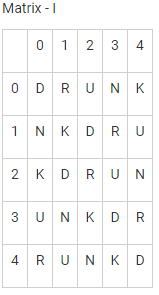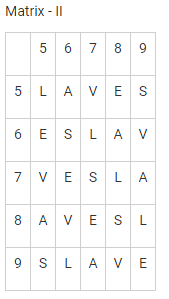Detailed Solution for Test: General Intelligence & Reasoning - 3 - Question 31

1) 33, 78, 40, 58 → DLRE

2) 21, 55, 13, 99 → DLRE

3) 44, 85, 34, 65 → DARE

4) 12, 67, 22, 87 → DLRE

Hence correct answer is 44, 85, 34, 65.

Test: General Intelligence & Reasoning - 3 - Question 32

From the given alternatives, select the word which can be formed using the letters of the given word.

CONTENTION

Detailed Solution for Test: General Intelligence & Reasoning - 3 - Question 32

Given word is CONTENTION

NATION – it can’t be formed.

TONIC – it can be formed.

OINTMENT – it can’t be formed.

STATION – it can’t be formed.

Hence, only TONIC is formed from given word.

Test: General Intelligence & Reasoning - 3 - Question 33

Identify the diagram that best represents the relationship among the classes given below:

Rat, Mammal, Eagle

Test: General Intelligence & Reasoning - 3 - Question 34

In the following question, the symbols %, #, & and @ are used with the following meaning as illustrated below:

L % M means L is son of M.

L # M means L is daughter of M.

L & M means L is brother of M.

L @ M means L is sister of M.

If D % B # C @ A, then how is A related to D?

Test: General Intelligence & Reasoning - 3 - Question 35

In the given diagram, Circle represents tall men, Square represents heavy men and Triangle represents generals. Which region represents generals who are heavy but not tall?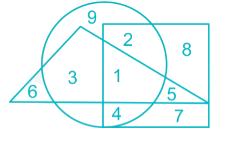Detailed Solution for Test: General Intelligence & Reasoning - 3 - Question 35

We require the part common between triangle and square, but not the circle. Therefore, the required region is denoted by 5.

Test: General Intelligence & Reasoning - 3 - Question 36

Arrange the following words as per order in the dictionary.

(1)  Acarpous
(2) Across
(3) Accede
(5) Accenture

Detailed Solution for Test: General Intelligence & Reasoning - 3 - Question 36

Solution: According to the Dictionary, the given words can be arranged as:

1. Acarpous

3. Accede

5. Accenture

2. Across

Hence, the correct order is 4, 1, 3, 5, 2

Test: General Intelligence & Reasoning - 3 - Question 37

Arrange the given words in the order in which they will be arranged in a dictionary and choose the one that comes second.

1. Triggered

2. Trenchant

3. Trembling

4. Trail

Detailed Solution for Test: General Intelligence & Reasoning - 3 - Question 37

First two letters of all the words are common i.e. ‘Tr’; hence we need to look at next letter.

As per third letter, ‘A’ comes before ‘E’, ‘E’ comes before ‘I’. Hence, Trail would come first, Trenchant and Trembling would come second and third in some order, while Triggered would come last.

For Trenchant and Trembling, we need to look at fourth letter as their first three letters are same. As per fourth letter, ‘M’ would come before ‘N’; hence Trembling would come before Trenchant.

Words in alphabetical order will be Trail, Trembling, Trenchant, Triggered.

Thus, the word that comes second is Trembling.

Test: General Intelligence & Reasoning - 3 - Question 38

If 'ARATHY' is coded as 'BSBUIZ' then 'SYSTEM' should be

Test: General Intelligence & Reasoning - 3 - Question 39

Directions:In making decisions about important questions, it is desirable to be able to distinguish between Strong arguments and weak arguments. Strong arguments are those, which are both important and directly related to the question. Weak arguments are those, which are of minor importance and also may not be directly related to the question or may be related to a trivial aspect of the question. The question below is followed by two arguments numbered I and II. You have to decide which of the arguments a strong argument is and which a Weak argument is.

Statement: Globalization has reduced patriotism of people and their love towards their motherland.

Arguments:

I. It leads to people leaving their own nations and serve other countries.

II. It makes the people forget about their own countries and makes them strive for the better.

Detailed Solution for Test: General Intelligence & Reasoning - 3 - Question 39

Both the arguments are weak. One person working in some other country does not mean serving the other country as one needs to pay proper taxes to the homeland. Patriotism has nothing to do with dreaming for the better. Thus option 4 is the correct answer.

Test: General Intelligence & Reasoning - 3 - Question 40

If ‘+’ means ‘÷’, ‘–’ means ‘x’, ‘÷’ means ‘–’ and ‘x’ means ‘+’, then,

42 ÷ 24 + 6 × 4 - 3 = ?

Detailed Solution for Test: General Intelligence & Reasoning - 3 - Question 40

42 ÷ 24 + 6 × 4 – 3 (Given Equation)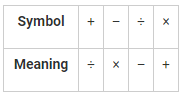⇒ Converting into Meaning symbols:

42 – 24 ÷ 6 + 4 × 3

Opening using BODMAS rule.

42 – 4 + 4 × 3

⇒ 42 – 4 + 12 = 54 – 4 = 50

Test: General Intelligence & Reasoning - 3 - Question 41

Directions: In the following question, two statements are given followed by two conclusions I and II. You have to consider the statements to be true even if they seem to be at variance from commonly which of the given conclusions, is any, follow from the given statements:

Statements:

1. Some idiots are clever.

2. All stupid’s are idiots.

Conclusions:

I. All idiots are stupid’s.

II. Some stupid’s can be clever

Test: General Intelligence & Reasoning - 3 - Question 42

Directions: Select the missing number from the given responses.

7          13           14

4          11           13

1           9             ?

Detailed Solution for Test: General Intelligence & Reasoning - 3 - Question 42

The numbers have been decreased by 3 in the first column, 2 in the second column and 1 in the third column.

Thus the missing number is 13 – 1 = 12

Test: General Intelligence & Reasoning - 3 - Question 43

Direction: In the following question, find the missing number from the given responses.

7          9          8

4          8          6

2          3          4

30       ?          52

Detailed Solution for Test: General Intelligence & Reasoning - 3 - Question 43

The relation is as shown below

7 × 4 = 28 + 2 = 30

8 × 6 = 48 + 4 = 52

Similarly,

9 × 8 = 72 + 3 = 75

Hence, the missing number is 75.

Test: General Intelligence & Reasoning - 3 - Question 44

In the given figure, circle stands for cultural club, triangle stands for sports club and rectangle stands for music club. Show who are in music club, as well as cultural club and not in sports club: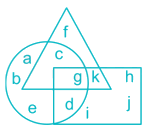Detailed Solution for Test: General Intelligence & Reasoning - 3 - Question 44

Given:-

Circle – Cultural Club

Triangle – Sports Club

Rectangle – Music Club

We need to find out those who are in Music and Cultural clubs but not in sports.

So, Circle + Rectangle – Triangle

So, ‘d’ is the one present in both circle and rectangle but not in triangle.

Hence, Option (B) is the right answer.

Test: General Intelligence & Reasoning - 3 - Question 45

How many triangles are there in the given figure?Test: General Intelligence & Reasoning - 3 - Question 46

Observe the figures and find a mirror image of the same from the alternatives given. Imagine that MN is a mirror.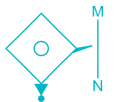Test: General Intelligence & Reasoning - 3 - Question 47

Find the figure from alternatives in which the question figure is hidden: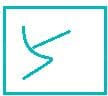Test: General Intelligence & Reasoning - 3 - Question 48

Which answer figure will complete the pattern in the question figure?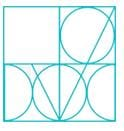Test: General Intelligence & Reasoning - 3 - Question 49

In the question below is given a statement followed by two conclusions numbered I and II. You have to assume everything in the statement to be true, then consider the two conclusions together and decide which of them logically follows beyond a reasonable doubt from the information given in the statement.

Statement: If mileage and looks are your criteria for buying a car, Renault’s Duster is your best bet.

Conclusions:

I. Renault Duster gives good mileage.

II. It is the best looking car of all times.

Detailed Solution for Test: General Intelligence & Reasoning - 3 - Question 49

The statement suggests that Renault Duster is being suggested to someone for whom mileage and looks are important attributes. Thus, it implies that the car gives good mileage. Hence, conclusion I follows.

The second conclusion cannot be deduced because the statement does not convey any information about comparison of the car with any other contemporary car in terms of looks. Thus this conclusion does not follow.

Test: General Intelligence & Reasoning - 3 - Question 50

Which of the following cube in the answer figure cannot be made based on the unfolded cube in the question figure?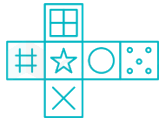## Mock Test Series for SSC JE Mechanical Engineering

3 videos|1 docs|55 tests
Information about Test: General Intelligence & Reasoning - 3 Page
In this test you can find the Exam questions for Test: General Intelligence & Reasoning - 3 solved & explained in the simplest way possible. Besides giving Questions and answers for Test: General Intelligence & Reasoning - 3 , EduRev gives you an ample number of Online tests for practice

## Mock Test Series for SSC JE Mechanical Engineering

3 videos|1 docs|55 tests(Scan QR code)